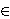### Ivica Gusić

Faculty of Chemical Engineering and Technology, University of Zagreb, Marulićev trg 19, 10000 Zagreb, Croatia
e-mail: igusic@fkit.hr

Abstract.   Let K be an algebraic number field, and let h(x)=x3+ax be a polynomial over K. We prove that there exists infinitely many bK such that the equation dy2=x3+ax+b has no solutions over K for infinitely many dK*/K* 2. The proof is based on recent results of B. Mazur and K. Rubin on the 2-Selmer rank in families of quadratic twists of elliptic curves over number fields. On the other side, it is known that if the parity conjecture is valid, then there exist a number field K and a cubic polynomial f irreducible over K, such that the equation dy2=f(x) has infinitely many solutions for each dK*.

2000 Mathematics Subject Classification.   11G05, 14G05.

Key words and phrases.   Elliptic curve, quadratic twist, 2-Selmer rank, number field.

Full text (PDF) (free access)

DOI: 10.3336/gm.46.2.05

References:

1. T. Dokchitser and V. Dokchitser, Elliptic curves with all quadratic twists of positive rank, Acta Arith. 137 (2009), 193-197.
MathSciNet     CrossRef

2. T. Dokchitser and V. Dokchitser, Root numbers and parity of ranks of elliptic curves, J. Reine Angew. Math. 2011, 658, 39-64.

3. A. Granville, Rational and integral points on quadratic twists of a given hyperelliptic curve, Int. Math. Res. Not. IMRN 2007, Art. ID 027, 24 pp. (2007).
MathSciNet

4. I. Gusić, A characterization of linear polynomials, J. Number Theory 115 (2005), 343-347.
MathSciNet     CrossRef

5. I. Gusić, Some applications of the abc-conjecture to the diophantine equation qym = f(x), Glas. Mat. Ser III., to appear.

6. B. Mazur and K. Rubin, Ranks of twists of elliptic curves and Hilbert's tenth problem, Invent. Math. 181 (2010), 541-575.
MathSciNet     CrossRef

7. J. Nakagawa and K. Horie, Elliptic curves with no rational points, Proc. Amer. Math. Soc. 104 (1988), 20-24.
MathSciNet     CrossRef

8. K. Ono and C. Skinner, Non-vanishing of quadratic twists of modular L-functions, Invent. Math., 134 (1998), 651-660.
MathSciNet     CrossRef

9. A. Schinzel, Polynomials with special regard to reducibility, Cambridge University Press, Cambridge, 2000.
MathSciNet

10. J. H. Silverman, The Arithmetic of Elliptic Curves, Graduate Texts in Mathematics, 106, Springer, Berlin, 1986.
MathSciNet